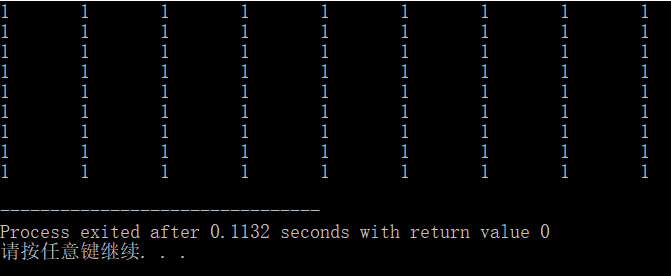• vector 数组初始化赋值 说明 总结c++ vector 一维数组，二维度数组的初始化赋值。 例子 #include<iostream> #include<vector> using namespace std; int main() { // 一维度，直接赋值 vector<...

vector 数组初始化赋值

说明

总结c++ vector 一维数组，二维度数组的初始化赋值。

例子

#include<iostream>
#include<vector>
using namespace std;

int main()
{
// 一维度，直接赋值
vector<int> arr = { 1, 2, 3 };
// 二维度直接赋值
vector<vector<char>> vec = {{'A', 'B', 'C', 'E'}, {'S', 'F', 'C', 'S'}, {'A', 'D', 'E', 'E'}};
// 一维，赋值。其中1为值，3为列数
vector<int> vecInt(3, 1);
// 二维，赋值。其中3为列数，vecInt为一维度数组
vector<vector<int>> vec2(3, vecInt);
cout << "endl" << endl;
}

展开全文c++
• 1. vector m1; 默认初始化，vector为空， size为0，表明容器中没有元素，而且 capacity 也...以另一个数组初始化此数组。 也可以使用等号连接。如：vector m1 = m2; 两种方式等价 ，m1 初始化为m2 的拷贝，m1与m...

1. vector m1;

默认初始化，vector为空， size为0，表明容器中没有元素，而且 capacity 也返回 0，意味着还没有分配内存空间。这种初始化方式适用于元素个数未知，需要在程序中动态添加的情况。

2. vector m1(m2);

以另一个数组初始化此数组。
也可以使用等号连接。如：vector m1 = m2;
两种方式等价 ，m1 初始化为m2 的拷贝，m1与m2都是vector数组即可。

3. vector m1 = {1,2,3};

vector m1 {1, 2, 3};

4. vector m1(m2.begin()+n,m2.end()-m);

范围拷贝，m1初始化后的值为m2.begin()+n到m2.end()-m的值，当n=m=0时，m1初始化为m2。
也可以使用普通数组初始化，如下：
int m2 = {1, 2, 3, 4, 5};
vector m1(m2, m2+5)

5. vector m1(2);

默认值初始化，m1中将包含2个元素，每个元素进行缺省的值初始化，对于int，也就是被赋值为0。

6. vector m1(2,1);

指定值初始化，m1被初始化为包含2个值为1的int

展开全文c++
• 二维vector数组初始化方法

千次阅读 2020-04-14 21:42:11
在用devcpp编译程序时发现，二维vector数组如果只定义的话，不指定元素个数也不进行初始化的时候会导致编译出错...二维数组初始化方法目前有以下两种： 第一种初始化方法（以元素类型为int为例） 初始化为1： `vect...

在用devcpp编译程序时发现，二维vector数组如果只定义的话，不指定元素个数也不进行初始化的时候会导致编译出错。
通常情况下，可以只提供vector对象容纳的元素数量而略去初始值。此时库会创建一个值初始化的元素初值，并把它赋给容器中的所有元素。这个初值由vector对象中元素的类型决定。
二维数组初始化方法目前有以下两种：

第一种初始化方法（以元素类型为int为例）

初始化为1：

vector<vector<int>> vec(row, vector<int> (col,1));

第二种初始化方法

初始化为1：

vector<vector<int>> vec;
vec.resize(row);
for(int i = 0; i < vec.size(); i++) {
vec[i].resize(col);
}
for(int i = 0; i < row; i++) {
for (int j = 0; j < col; j++) {
vec[i][j] = 1;
}
}

完整程序

#include <iostream>
#include <vector>
using namespace std;

void print(const vector<vector<int>> vec)
{
for(int i = 0; i < vec.size(); i++) {
for(int j = 0; j < vec.size(); j++) {
cout<<vec[i][j]<<"\t";
if(j == vec.size() - 1)
cout<<endl;
}
}
}
int main()
{
int row = 9;
int col = 9;
//vector<vector<int>> vec(row, vector<int> (col,1));
vector<vector<int>> vec;
vec.resize(row);
for(int i = 0; i < vec.size(); i++) {
vec[i].resize(col);
}
for(int i = 0; i < row; i++) {
for (int j = 0; j < col; j++) {
vec[i][j] = 2;
}
}
print(vec);
}

效果展开全文• ** 一、定义一个一维vector数组v1，数组大小为n，初始值为0** 初试化数组长度 ...初始化数组大小和值 vector<int> v1(m, 0); //size = 100; every item = 0 拷贝vector数组 vector<...

一、定义一个一维vector数组v1，数组大小为n，初始值为0

1. 初试化数组长度
const int m = 100;
vector<int> v1(m);		//size = 100

1. 初始化数组大小和值
vector<int> v1(m, 0);		//size = 100;  every item = 0

1. 拷贝vector数组
vector<int> a(m, 0);
vector<int> b(a);

二、定义一个m*n的二维vector数组v2，初始值为0

1. 直接初始化
vector<vector<int> > v2(m, vector<int>(n, 0));

1. 利用resize()函数
vector<vector<int> > v2;
v2.resize(m);		//row size = m
for(int i=0; i<m; i++) {
v2[i].resize(n);			//column size = n
}

展开全文• C++ Vector数组初始化(一维，二维)

千次阅读 多人点赞 2019-11-06 11:10:48
Vector数组快速声明与初始化 一维 以int类型为例子 设置数组长度的默认初始vector<int> data(N); N为默认数组长度，默认初始值为0 设置数组长度的给定值初始vector<int> data(N,value); N为...
• 一.概念 vector<type> 容器是包含 type 类型元素的序列容器。...二.vector数组的创建 vector<type> name; type:指数组类型，例如int,float,double,string等等。 name: 创建的数组的名称，想取什么取c++
• vector常用方法 assign() 对Vector中的元素赋值 void assign( input_iterator start, input_iterator end ); //voidc++ 初始化 数组 vector
• c++基础之vector、数组初始化

万次阅读 多人点赞 2018-08-30 10:11:31
默认定义了一个size为5初始值全为0的vector 定义了size为5的初始值全为1的vector v2 如果要指定vector中的每个值，可以通过先定义一个...下面来看看二维vector初始化 可以看到vv初始化为5个size为0的vecto...
• vector> #include<iterator> using namespace std; int main(void){ int array={ 0,1,2,3,4,5,6,7,8,9 }; vector<int>v(begin(array),end(array)); for(auto i=v.begin();i<v.end()c++
• 使用数组初始化vector对象

千次阅读 2018-06-14 09:18:36
允许使用数组初始化vector对象。要实现这一目的，只需指明要拷贝区域的首元素地址和尾后地址即可。int arr={0,1,2,3,4,5,6};vector&lt;int&gt; vec(begin(arr),end(arr));...
• 可是，如果我们只知道行或者列其中的一个数量，可以进行动态数组初始化吗？  答案是可以的。如果我们知道行数，那么初始化可以如此写：vector>Name(row,vector(0))。这样的含义是：行数为row，列数为0。那如何对这...c++ vector 动态数组 初始化
• 如： int values; int count = 256; hidl_vec<int32_t> v(std::vector<int32_t>(values, values + count));...vector 使用数组初始化时，为左闭右开，意思是count=256,则std::vector<...c++
• 数组初始化方式： 2.vector六种初始化方式： 3.string初始化方式： 4.string也能push_back()和pop_back() 5.string的插入删除操作 1.数组初始化方式： https://www.cnblogs.com/yyzyxy/p/10336480.html 2.vector六种...c++
• 初始化vector数组以及使用

千次阅读 2020-07-22 21:00:49
vector数组初始化： vector month = { 1,2,3,4,5,6,7,8,9,10,11,12 } vector month = { 1,2,3,4,5,6,7,8,9,10,11,12 } vector< int > month { 12 } 像这样的声明会有歧义，究竟是0位置有一个元素12还是大小...c++
• 原文：... 在LeetCode刷算法的时候发现，使用vector<vector<int>> 时如果声明时不初始化，会报空指针引用错误：reference binding to null pointer of type 'value_type'。 根据《...
• vector二维数组初始化

千次阅读 2018-12-05 18:28:37
int main() { vector<vector<int> >num = { {1,1,1,0,1,1},{1,0,1,1,1,1},{1,1,1,0,0,1},{1,0,1,0,0,1},{1,1,1,1,1,1} }; ...
• C++：vector二维数组初始化

千次阅读 2021-03-31 15:12:37
在刷 LeetCode 的时候需要初始化创建一个行大小为r、列大小为c的 vector 二维数组，具体的实现方法如下： vector<vector<int>> num(r, vector<int>(c)); 初始化创建r个类型为vector<int>，...c++ 开发语言
• c++允许用数组初始化vector对象，不过要指明要拷贝区域的首元素地址和尾元素的地址 程序一 #include #include<vector> int main() { using std :: vector ; using std ::begin; using std ...
• C++ 使用数组初始化Vector

千次阅读 2015-08-02 16:11:56
vector初始化常常需要一个...为了不用一个元素与个元素的 push_back 到 vector 之中，本文拟采用指针赋值的方式，一次性操作以实现数组vector初始化，相见代码如下。 #include #include   using name数组 STL vector 初始化 声明
• Do I have to push my elements one by one? I tried something likeString[]...array=...Vector vector = new Vector(array);but my eclipse marks this as an error.解决方案Vector doesn't have a constructor th...
• 方法1：nt N=5, M=6; vector<vector<int> > Matrix(N); for(int i =0; i(); i++){ Matrix[i].resize(M); } 方法2：#include <iostream> #include<vector> #include #include usin...